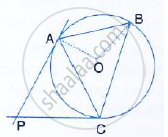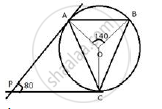Share

In the Given Figure, O is the Centre of the Circumcircle Abc. Tangents at a and C Intersect at P. Given Angle Aob = 140° and Angle Apc = 80°; Find the Angle Bac. - ICSE Class 10 - Mathematics

ConceptTangent Properties - If a Line Touches a Circle and from the Point of Contact, a Chord is Drawn, the Angles Between the Tangent and the Chord Are Respectively Equal to the Angles in the Corresponding Alternate Segments

Question

In the given figure, O is the centre of the circumcircle ABC. Tangents at A and C intersect at P. Given angle AOB = 140° and angle APC = 80°; find the angle BAC.SolutionJoin OC.
Therefore, PA and PA are the tangents
∴ OA  ⊥ PA and OC ⊥  PC
∠APC+ ∠ AOC  =  180°
⇒ 80° + ∠AOC =  180°
⇒ ∠AOC = 100°
∠ BOC = 360° - ( ∠ AOB + ∠AOC)
∠ BOC ≅  360°  - (140° + 100° )
∠B = 360° - 240° =120°
Now, arc BC subtends  ∠BOC at the centre and ∠BAC at the remaining part of the circle
∴ ∠BAC = 1/2 ∠BOC
∠BAC =1/2  × 120° = 60°

Is there an error in this question or solution?

Video TutorialsVIEW ALL 

Solution In the Given Figure, O is the Centre of the Circumcircle Abc. Tangents at a and C Intersect at P. Given Angle Aob = 140° and Angle Apc = 80°; Find the Angle Bac. Concept: Tangent Properties - If a Line Touches a Circle and from the Point of Contact, a Chord is Drawn, the Angles Between the Tangent and the Chord Are Respectively Equal to the Angles in the Corresponding Alternate Segments.
S